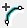# Liner Type: Geosynthetic

To define the support properties of reinforcement materials such as geogrids or geotextiles:

1. Select Define Linersfrom the toolbar or the Properties menu.
2. Select Liner Type = Geosynthetic.
3. Enter the properties described below. Properties are specified per unit width of material.

NOTE: for an overview of modeling Geosynthetics in RS2, see the Define Geosynthetics topic. This discusses the different ways of modeling Geosynthetic support, including the properties of the Geosynthetic/soil interface.

## Tensile Modulus

The Tensile Modulus represents the elastic response of the Geosynthetic to a tensile load. It is equal to the slope of a force versus strain graph, per unit width of material. Strain is the elongation strain measured in the direction of the applied tensile force.

#### Strength Parameters

The following Strength Parameters are applicable for a Geosynthetic.

Material Type: Elastic

If the Material Type = Elastic, then the strength of the Geosynthetic is not considered (i.e. there will be no upper limit to the tensile load which can be sustained by the Geosynthetic). The only property of the Geosynthetic which will be used in the analysis is the Tensile Modulus.

Material Type: Plastic

If the Material Type = Plastic, then you may enter values of Tensile Strength (peak) and Tensile Strength (residual).

• Peak Tensile Strength - determines the maximum load (force) which can be sustained by the Geosynthetic, per unit width of material.
• Residual Tensile Strength - if the peak Tensile Strength is reached, then the Geosynthetic will fail in tension, and the strength (force in the Geosynthetic) will revert to the residual Tensile Strength. If the Geosynthetic has no residual tensile strength then enter zero for this parameter.

## Axial Strain

This option allows users to assign axial strain to simulate liner contraction/expansion. In terms of the sign convention, negative input parameter (-) indicates contraction, and positive (+) represents expansion. The liner axial strain can be staged, using the Stage Geosynthetic Properties option in the dialog.

## Stage Geosynthetic Properties

The properties of a Geosynthetic can be modified at different stages of a multi-stage model, with the Stage Geosynthetic Properties option. This could be used, for example, to simulate a decrease in Geosynthetic strength or stiffness over time.

The Geosynthetic properties can be increased or decreased by user-defined factors at different stages. See the Stage Liner Properties topic for details about staging Geosynthetic properties.

## Flexural Properties of a Geosynthetic

By definition in RS2, a Geosynthetic has zero flexural rigidity. If you need to model a Geosynthetic with flexural rigidity (e.g. a stiff geogrid), then you should model this as a regular liner (e.g. Liner Type = Standard Beam), and enter appropriate properties to simulate the flexural behaviour.